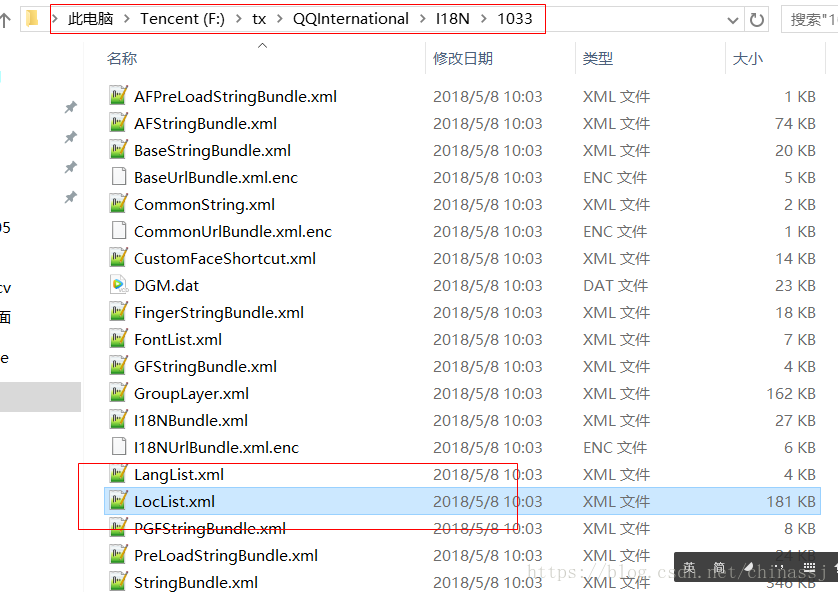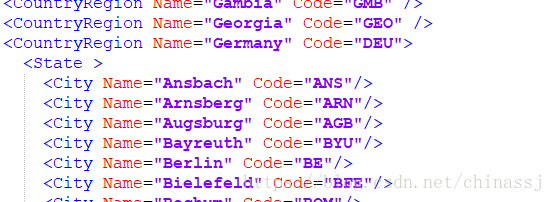# 如何获取轻松世界国家、省/州、城市名称的数据库

V2EX上高手一语点破穹天。

<Location>

<CountryRegion Name="中国" Code="1">

<State Name="北京" Code="11">

<City Name="东城" Code="1" />

<City Name="西城" Code="2" />

<City Name="朝阳" Code="5" />

<City Name="丰台" Code="6" />

<City Name="石景山" Code="7" />

<City Name="海淀" Code="8" />

<City Name="门头沟" Code="9" />

<City Name="房山" Code="11" />

<City Name="通州" Code="12" />

<City Name="顺义" Code="13" />

<City Name="昌平" Code="21" />

<City Name="大兴" Code="24" />

<City Name="平谷" Code="26" />

<City Name="怀柔" Code="27" />

<City Name="密云" Code="28" />

<City Name="延庆" Code="29" />

</State>

code都是按照ISO3166标准写的，极易导入数据库。。。## virtualbox 备份文件直接注册为虚拟机出错:which has the same UUID as an existing virtual machine.

virtualbox 备份文件直接注册为虚拟机出错:which has the same UUID as an existing virtual machine.